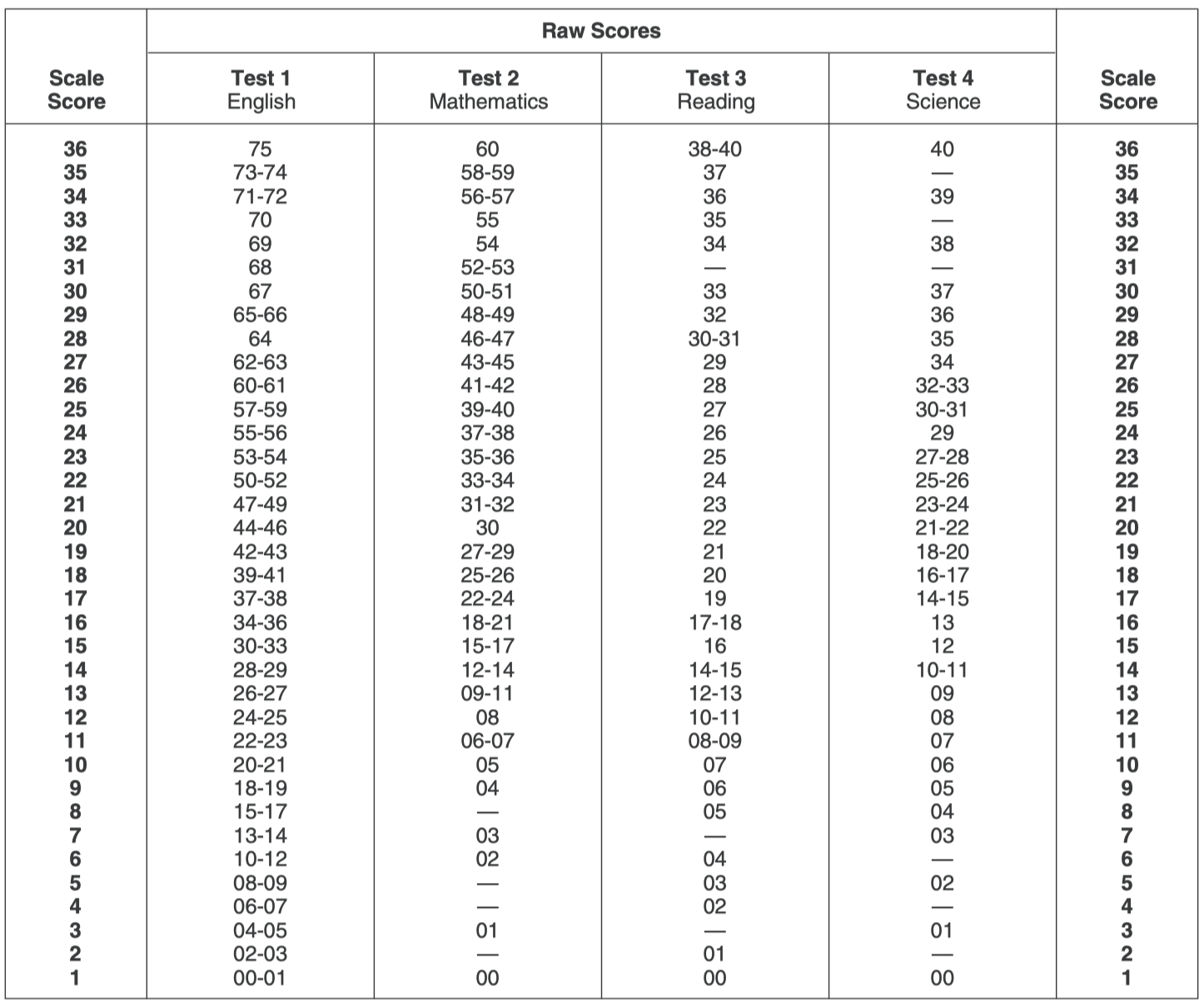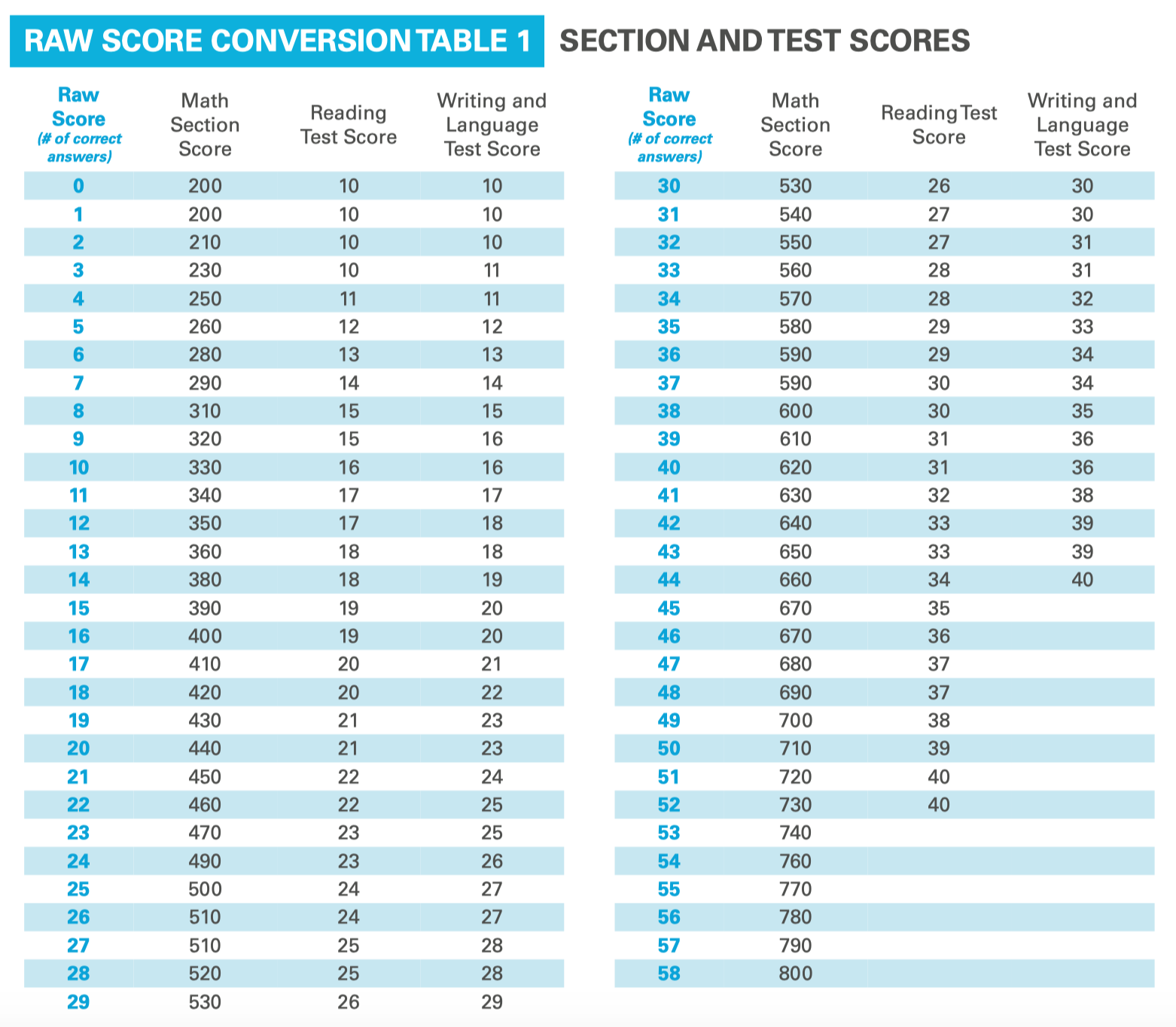# Understanding your SAT/ACT test results: scaled scores & percentilesWhile your SAT/ACT score report provides a lot of helpful feedback on your test performance, it can be difficult to make sense of the numbers. Both the SAT and ACT use scaled scores and percentiles to show how you did. It’s important to understand how the scoring works so that you can use your test prep energy most effectively.

Scaled scores and percentiles function differently than the grades students may be accustomed to receiving on tests in high school: traditional tests count your correct answers and divide that number by the total number of questions on the test. For example, if you answer 44 out of 58 questions correctly on a test in school, 44/58 would give you a grade of 76% or a C.

The SAT and ACT handle scores in different ways. Scaled scores and percentiles take into account not only how you did but also how the other test-takers did on the same test. Here's how it all works.

#### Scaled scores

To calculate your individual section scores, the College Board and ACT start with the number of questions you answer correctly, also known as your raw score. If you answer 44 out of 58 questions correctly on a section of the test, your raw score for that section is 44.

Your raw score for each section is then converted into a scaled score. On the ACT, each scaled score is a number out of 36.

• You'll get a scaled score out of 36 for each of the four multiple-choice sections: English, Math, Reading, and Science.
• Those four scaled scores are averaged (added up and divided by 4) to give you your composite score. Example: if I earn scaled scores of 32 in English, 28 in Math, 31 in Reading, and 29 in Science, my composite score is 30 because (32+28+31+29)/4 = 30.

The ACT score conversion table looks like this:On the SAT, your scaled scores are calculated differently for the verbal sections than for the math sections.

• You'll receive scaled scores out of 40 for the reading and writing sections. Your reading and writing scaled scores are then added together and multiplied by 10 to give you a scaled verbal score out of 800. Example: if I earn scaled scores of 33 in reading and 35 in writing, my verbal score becomes (33+35)x10 = 680.
• Your raw score for the math sections is converted directly into a scaled score out of 800.
• Your verbal and math scores are added together to give you a total score out of 1600. Example: if I earn 680 on the verbal sections and 650 on the math sections, my total score is 680+650 = 1330.

The SAT score conversion table looks like this:Keep in mind that scaled scores are adjusted for slight differences in difficulty across test administrations. If the test-takers on a given day perform a little better as a whole, the curve will be harsherstudents will be penalized more for each incorrect answer. If everyone performs slightly worse, the curve will be more forgiving—students will have more wiggle room to answer more questions incorrectly. That's why, for example, a raw math score of 44 on the SAT will usually result in a scaled score in the mid-600s but could end up as low as 620 or as high as 680 on a given day.

#### Percentiles

Your percentile rank represents the percentage of students whose score is equal to or lower than yours. An SAT math score of 650, for example, puts you in the 90th percentile, meaning 90% of test-takers earned scores at or below yours.

On the SAT, two percentiles will show up on your official score report. The Nationally Representative Sample Percentile represents all U.S. students in grades 11 and 12, regardless of whether those students typically take the SAT. The SAT User Percentile is based on the actual scores of high school students who took the SAT during the previous academic year. You can take a deeper look at those numbers here.

On the ACT, you'll also see two separate percentiles: a U.S. Rank and a State Rank. Your ranks represent the percentages of recent high school graduates in the U.S. and recent high school graduates in your state who took the ACT and earned scores equal to or lower than yours. You can take a deeper look at those numbers here.

#### Why does this matter?

Understanding how to interpret your scores puts you in a better position to use your test prep energy effectively.

• You may realize it'll be easier for you to improve your scaled score in one area than in another, resulting in greater score gains in a shorter period of time.
• Furthermore, knowing what the percentiles mean can help you set realistic goals that make sense in the context of your long-term plans.

Still confused about what your scores mean for you? We're here to help! The team of experts at ArborBridge is happy to help you analyze your results, so don't hesitate to reach out.

The recommendations above are general suggestions. If you have specific questions, reach out to our experts here. We’re happy to help in any way we can.